# Measuring Cups

## 1 Cup

"1 Cup" is equal to 8 fluid ounces in US Standard Volume. It is a measure used in cooking.

A Metric Cup is slightly different: it is 250 milliliters (which is about 8.5 fluid ounces).

Ounces
(at 8 oz per cup)
Tablespoons
(at 16 Tbs per cup)
milliliters
(at 250 ml per cup)
¼ cup 2 oz 4 Tbs 63 ml
½ cup 4 oz 8 Tbs 125 ml
¾ cup 6 oz 12 Tbs 188 ml
1 cup 8 oz 16 Tbs 250 ml
1 ¼ cup 10 oz 20 Tbs 313 ml
1 ½ cup 12 oz 24 Tbs 375 ml
1 ¾ cup 14 oz 28 Tbs 438 ml
2 cups 16 oz 32 Tbs 500 ml
4 cups 32 oz 64 Tbs 1000 ml (1 liter)
8 cups 64 oz 128 Tbs 2000 ml (2 liters)

(In italics: not exact)

## Measuring Cups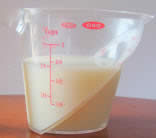Measuring cups are used to measure liquids, or powders like flour or sugar.

As we fill up the measuring cup, we need to look at which number it is filled to.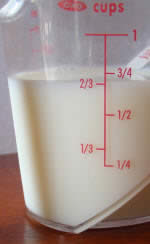Here we have 2/3 cup (two-thirds of a cup) of milk.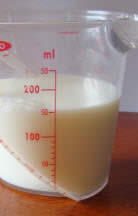And they can also measure milliliters (ml):

Here we have 150 ml.

It doesn't say "150" ... it says "50" ... but it is half-way between 100 and 200 so we can figure out it is 150 ml.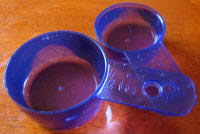There are also special ones designed to hold an exact amount, such as these 1/2 cup and 1/4 cup measures.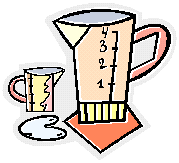They can also hold more than "1 Cup" of liquid.

This measuring cup holds up to 4 cups of liquid. If the liquid goes all the way to the 3, then there are 3 cups of liquid. Between the numbers we find fractions of a cup.

2 cups of a liquid is the same thing as 1 pint.  So this measuring cup also holds 2 pints (4 cups equals 2 pints).

1917, 1918